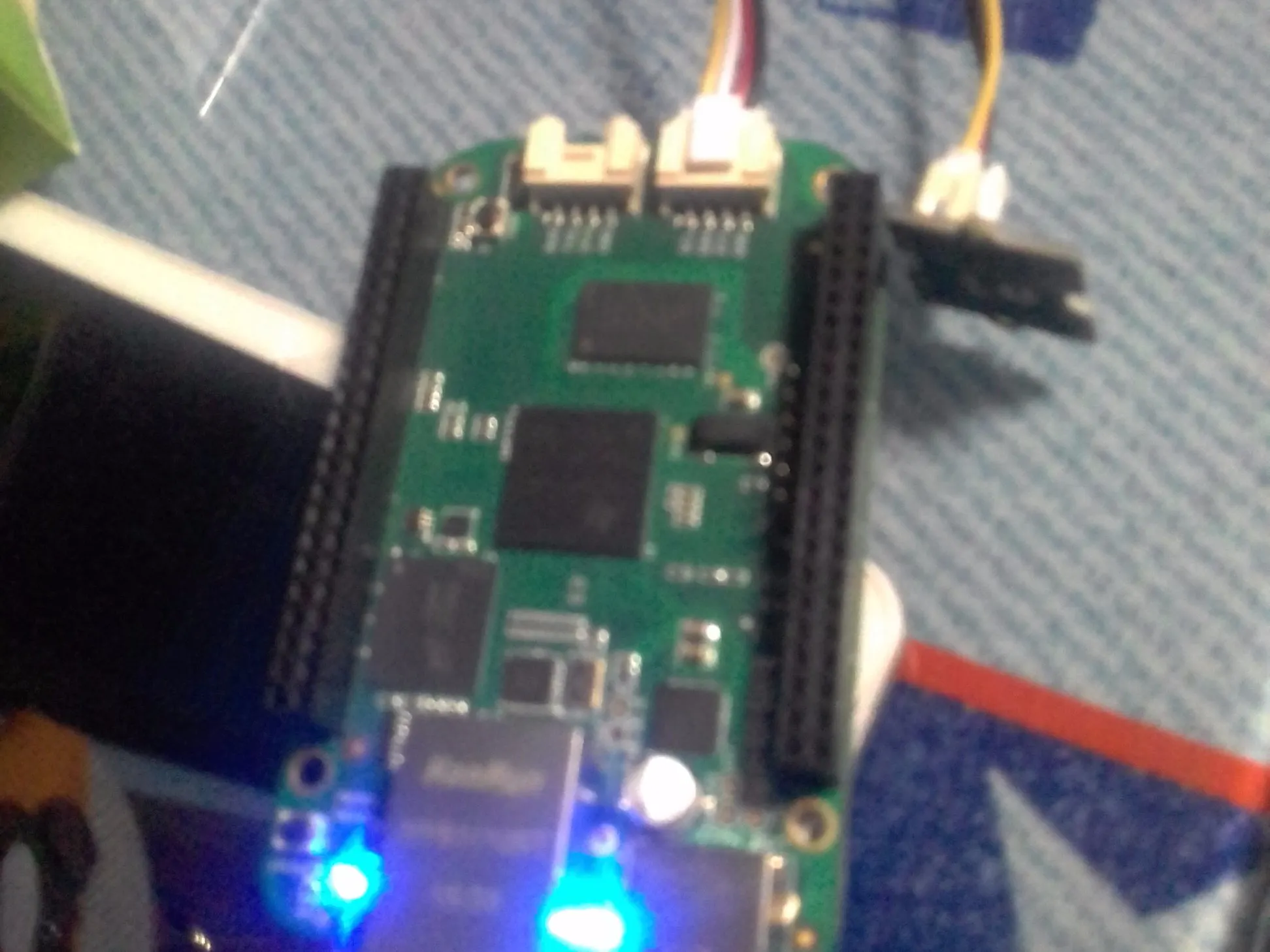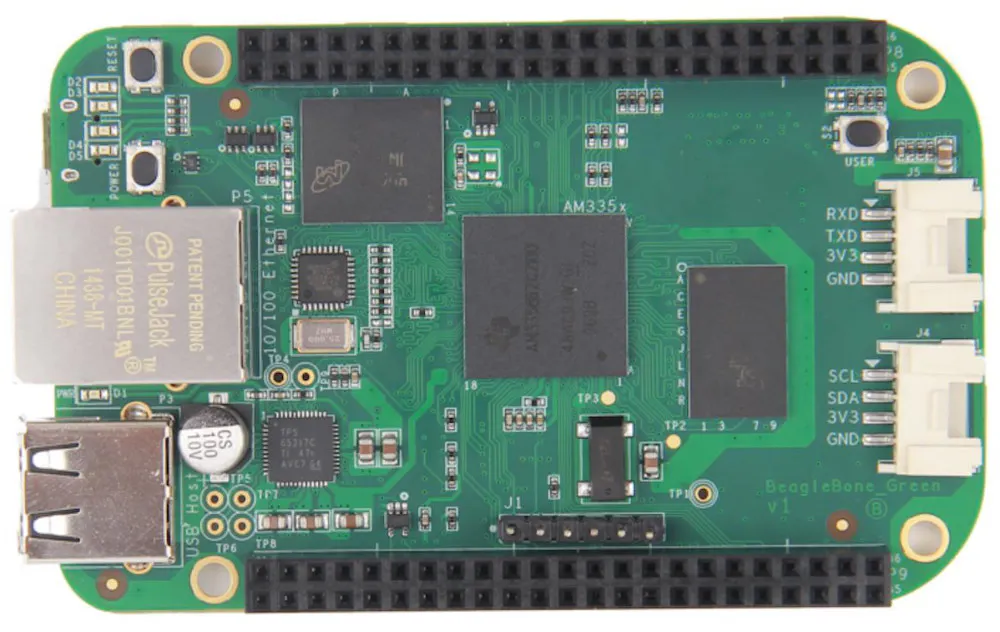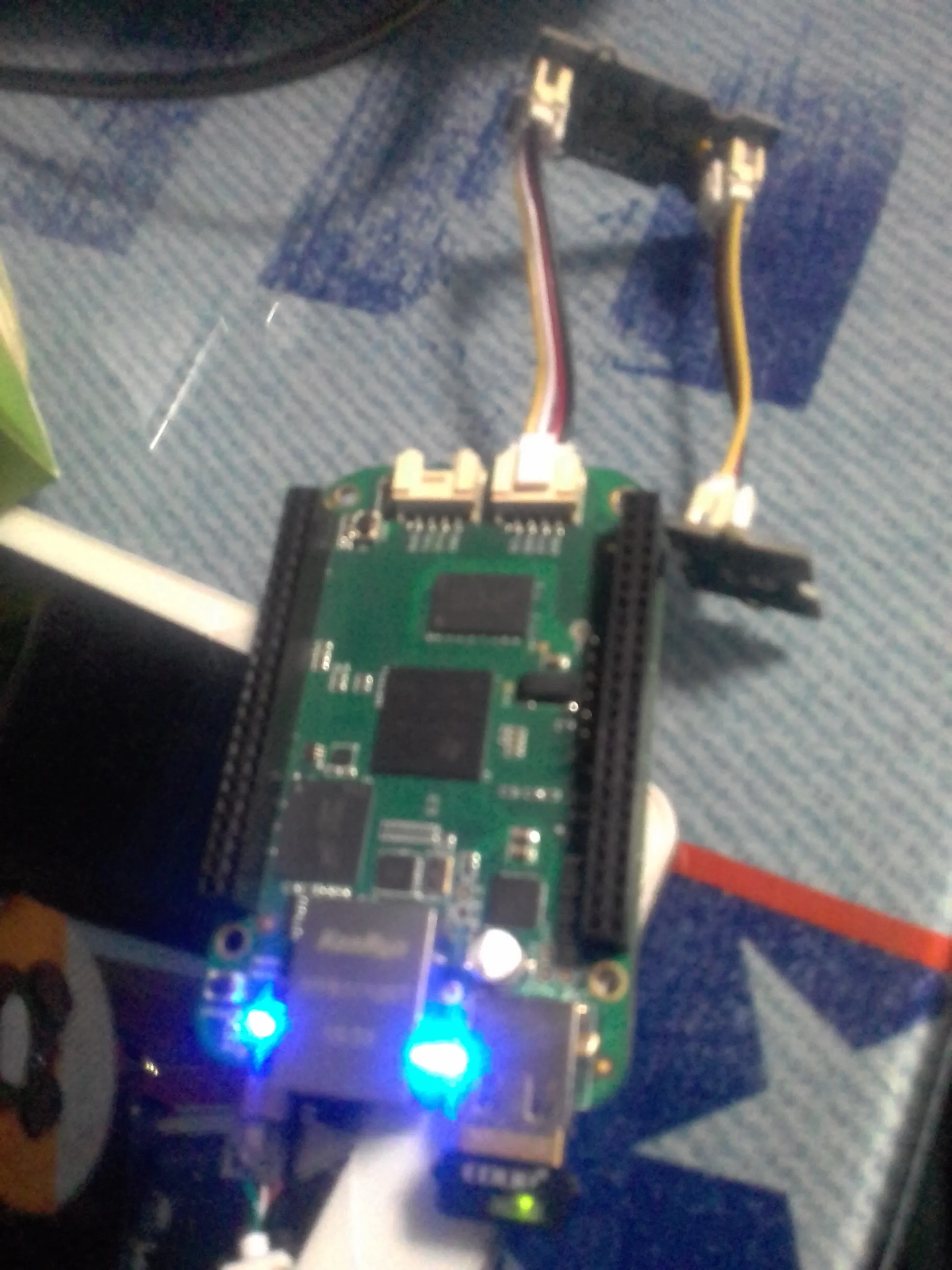# BeagleBone Green Temperature Monitor on Artik Cloud

Publish Grove Temperature Sensor values collected by a BeagleBone Green to Artik Cloud.

IntermediateFull instructions provided2 hours997## Things used in this project

### Hardware componentsBeagleBoard.org SeeedStudio BeagleBone Green
×1
×1
 Seeed Grove Temperature Sensor
×1

### Software apps and online servicesSamsung ARTIK Cloud for IoT

## Schematics

### 20160928_000644.jpg## Code

Python
```import time
import math

BUZZER = "P9_22"            # GPIO P9_22
GPIO.setup(BUZZER, GPIO.OUT)
# The threshold to turn the buzzer on 28 Celsius
THRESHOLD_TEMPERATURE = 28
#   The argument in the read_temperature() method defines which Grove board(Grove Temperature Sensor) version you have connected.
#   Defaults to 'v1.2'. eg.
#       temp = read_temperature('v1.0')          # B value = 3975
#       temp = read_temperature('v1.1')          # B value = 4250
#       temp = read_temperature('v1.2')          # B value = 4250
"Read temperature values in Celsius from Grove Temperature Sensor"
# each of the sensor revisions use different thermistors, each with their own B value constant
if model == 'v1.2':
bValue = 4250  # sensor v1.2 uses thermistor ??? (assuming NCP18WF104F03RC until SeeedStudio clarifies)
elif model == 'v1.1':
bValue = 4250  # sensor v1.1 uses thermistor NCP18WF104F03RC
else:
bValue = 3975  # sensor v1.0 uses thermistor TTC3A103*39H

total_value = 0
for index in range(20):
total_value += sensor_value
time.sleep(0.05)
average_value = float(total_value / 20)
# Transform the ADC data into the data of Arduino platform.
sensor_value_tmp = (float)(average_value / 4095 * 2.95 * 2 / 3.3 * 1023)
resistance = (float)(1023 - sensor_value_tmp) * 10000 / sensor_value_tmp
temperature = round((float)(1 / (math.log(resistance / 10000) / bValue + 1 / 298.15) - 273.15), 2)
return temperature
# Function: If the temperature sensor senses the temperature that is up to the threshold you set in the code, the buzzer is ringing for 1s.
# Hardware: Grove - I2C ADC, Grove - Temperature Sensor, Grove - Buzzer
# Note: Use P9_22(UART2_RXD) as GPIO.
# Connect the Grove Buzzer to UART Grove port of Beaglebone Green.
# Connect the Grove - I2C ADC to I2C Grove port of Beaglebone Green, and then connect the Grove - Temperature Sensor to Grove - I2C ADC.
if __name__ == '__main__':
while True:
try:
# Read temperature values in Celsius from Grove Temperature Sensor
# When the temperature reached predetermined value, buzzer is ringing.
print "temperature = ", temperature
except IOError:
print "Error"
```

### post.py

Python
try postin using rest
```import requests
import json
import time

ts = int(time.time())

url = 'https://api.artik.cloud/v1.1/messages'

data = {
'sdid': '385261a597fd4e808562fde4a87dee3f',
'type': 'message',
'ts': '1475160627',
'data': {'TEMPERATURE':36}
}
params = {}

print response.status_code
print response.text
```

### send-temp.py

Python
Send the temperature value to Artik Cloud
```import time
import math

BUZZER = "P9_22"            # GPIO P9_22
GPIO.setup(BUZZER, GPIO.OUT)
THRESHOLD_TEMPERATURE = 28
"Read temperature values in Celsius from Grove Temperature Sensor"
# each of the sensor revisions use different thermistors, each with their own B value constant
if model == 'v1.2':
bValue = 4250  # sensor v1.2 uses thermistor ??? (assuming NCP18WF104F03RC until SeeedStudio clarifies)
elif model == 'v1.1':
bValue = 4250  # sensor v1.1 uses thermistor NCP18WF104F03RC
else:
bValue = 3975  # sensor v1.0 uses thermistor TTC3A103*39H
total_value = 0
for index in range(20):
total_value += sensor_value
time.sleep(0.05)
average_value = float(total_value / 20)
# Transform the ADC data into the data of Arduino platform.
sensor_value_tmp = (float)(average_value / 4095 * 2.95 * 2 / 3.3 * 1023)
resistance = (float)(1023 - sensor_value_tmp) * 10000 / sensor_value_tmp
temperature = round((float)(1 / (math.log(resistance / 10000) / bValue + 1 / 298.15) - 273.15), 2)
return temperature

if __name__ == '__main__':

while True:
try:
# Read temperature values in Celsius from Grove Temperature Sensor
# When the temperature reached predetermined value, buzzer is ringing.
print "temperature = ", temperature
import requests
import json
import time

ts = int(time.time())
print "time = ",ts

url = 'https://api.artik.cloud/v1.1/messages'

data = {
'sdid': '385261a597fd4e808562fde4a87dee3f',
'type': 'message',
'ts': ts,
'data': {'TEMPERATURE':temperature}
}
params = {}

print response.status_code

time.sleep(1)

except IOError:
print "Error"
```

## Credits

### Laurence Daxes

3 projects • 27 followers
Systems Engineer Bachellor, I Love technology, and IoT World https://youtube.com/c/DaxesHacks